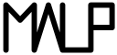Multi-ApplicationOnlineProfiling  2.1
Bit Array

This is a bit array data structure implementation. More...

## Data Structures

struct  Bit_Array
Defines a bit array. More...

## Functions

static uint64_t Bit_Array_size (struct Bit_Array *ba)
Getter on the array size. More...

void Bit_Array_init (struct Bit_Array *ba, uint64_t size)
Bit_Array initializer. More...

void Bit_Array_release (struct Bit_Array *ba)
Bit_Array deinitializer. More...

void Bit_Array_replicate (struct Bit_Array *dest, struct Bit_Array *src)
Copies a Bit_Array into another. More...

void Bit_Array_dump (struct Bit_Array *ba)
Prints a Bit_Array on standard output. More...

static void Bit_Array_set (struct Bit_Array *ba, uint64_t key, uint8_t value)
Sets a bit in a Bit_Array. More...

static uint8_t Bit_Array_get (struct Bit_Array *ba, uint64_t key)
Gets a bit in a Bit_Array. More...

## Variables

Masks used to get and set bits. More...

Masks used to unset bits. More...

## Detailed Description

This is a bit array data structure implementation.

## Function Documentation

 void Bit_Array_dump ( struct Bit_Array * ba )

Prints a Bit_Array on standard output.

Parameters
 ba the Bit_Array to print

Definition at line 68 of file Bit_Array.c.

Here is the call graph for this function: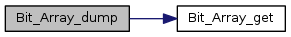static uint8_t Bit_Array_get ( struct Bit_Array * ba, uint64_t key )
inlinestatic

Gets a bit in a Bit_Array.

Parameters
 ba the Bit_Array where to get the bit key the index of the bit to get
Returns
the value of the bit (3 if out of bound)

Definition at line 167 of file Bit_Array.h.

Here is the caller graph for this function: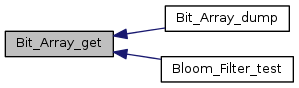void Bit_Array_init ( struct Bit_Array * ba, uint64_t size )

Bit_Array initializer.

Parameters
 ba the Bit_Array to initialize size the number of bits wanted fot ba

Definition at line 23 of file Bit_Array.c.

Here is the caller graph for this function: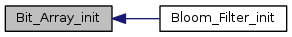void Bit_Array_release ( struct Bit_Array * ba )

Bit_Array deinitializer.

Parameters
 ba the Bit_Array to deinitialize

Definition at line 41 of file Bit_Array.c.

Here is the caller graph for this function: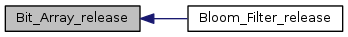void Bit_Array_replicate ( struct Bit_Array * dest, struct Bit_Array * src )

Copies a Bit_Array into another.

Parameters
 dest the destination Bit_Array src the source Bit_Array
Warning
Both src and dest must be initialized at the same size before copying.

Definition at line 55 of file Bit_Array.c.

Here is the caller graph for this function: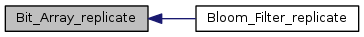static void Bit_Array_set ( struct Bit_Array * ba, uint64_t key, uint8_t value )
inlinestatic

Sets a bit in a Bit_Array.

Parameters
 ba the Bit_Array where to set the bit key the index of the bit to set value the value to set

Definition at line 140 of file Bit_Array.h.

Here is the caller graph for this function: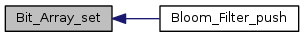static uint64_t Bit_Array_size ( struct Bit_Array * ba )
inlinestatic

Getter on the array size.

Parameters
 ba the array
Returns
the number of bits ba contains

Definition at line 101 of file Bit_Array.h.

## Variable Documentation

static
Initial value:
= {
1,
2,
4,
8,
16,
32,
64,
128
}

Masks used to get and set bits.

It is binary anded/ored with the buffer to get/set the bit value :

Example in 4 bits : 0001 0010 0100 1000

Definition at line 51 of file Bit_Array.h.

static
Initial value:
= {
254,
253,
251,
247,
239,
223,
191,
127
}